Time: 3 Hours                                                                                                     Max. Marks: 100

NOTE: There are 9 Questions in all.

·      Question 1 is compulsory and carries 20 marks. Answer to Q. 1. must be written in the space provided for it in the answer book supplied and nowhere else.

·      Out of the remaining EIGHT Questions answer any FIVE Questions. Each question carries 16 marks.

·      Any required data not explicitly given, may be suitably assumed and stated.

Q.1       Choose the correct or best alternative in the following:                                         (2x10)

a.       Which refresh procedure is used in TV sets to avoid flicker?

(A)  Horizontal retrace                         (B)  Vertical retrace

(C)    Interlaced refresh                         (D)  None of the above

b.      This is one of the side effects of scan conversion.

(A)  Aliasing                                        (B)  Tilting

(C)  Filling                                           (D)  None of the above

c.   The new coordinates of the point (2, -4) after rotation by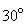about the origin is

(A)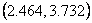(B)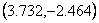(C)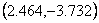(D)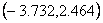d.   Let R be the rectangular window whose lower left hand corner is at L(-3, 1) and upper right hand corner is at R(2, 6); and the syntax of endpoint code from left to right be : top below right left.  The endpoint code of the point      (3, 3) is

(A)     0000                                            (B)  1000

(C) 1001                                            (D)  0010

e.   The number of visibly distinct dots that can be displayed in a given area of the screen is called

(A) coordinates.                                  (B)  bit precision.

(C) screen resolution.                           (D)  pixels.

f.    Intra and Inter compression scheme is used in

(A) Text.                                             (B) Images.

(C) Video.                                           (D) All of these.

g.   This method blurs the image for getting a gross representation of objects of interest

(A)  Spatial averaging                          (B)  Sharpening

(C)  Edge detection                             (D)  Transform

h.   To center the Fourier transform of an image, the image should be multiplied by

(A)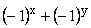(B)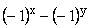(C)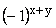(D)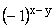i.    Four color printing refers to the following colors

(A)   Red, Green, Blue, Yellow             (B) Cyan, Magenta, Yellow, Red

(C) Hue, Saturation, Intensity, Black    (D) Cyan, Magenta, Yellow, Black

j.    Region splitting and merging technique has a convenient representation in the form of a so-called

(A)  Binary tree                                   (B)  Ternary tree

Answer any FIVE Questions out of EIGHT Questions.

Each question carries 16 marks.

Q.2     a.   Briefly explain back face visible surface detection algorithm.                                (6)

b.   How does a color CRT monitor function?  Explain the two basic techniques for producing color displays with a CRT.                                         (10)

Q.3     a.   Indicate which raster locations would be chosen by Bresenham’s algorithm when scan converting a line from screen coordinate (1, 1) to screen coordinate (8, 5). Show the intermediate steps also.       (8)

b.   Explain with an example the composite transformation required for magnifying a square while keeping its center fixed.  Obtain the matrix description of this composite transformation.       (8)

Q.4     a.   What are clipping algorithms?  What is line clipping?  Explain the steps involved in Cohen Sutherland line clipping algorithm.                                (8)

b.   In a three-dimensional coordinate system, tilting is defined as a rotation about the x-axis followed by a rotation about the y-axis.  Find the tilting matrix.  Does the order of performing the rotation matter? (8)

Q.5     a.   Explain the steps involved in a general three-dimensional transformation sequence from modelling coordinates to final device coordinates.                   (8)

b.   What is projection?  Compare and contrast the two basic projection methods.  Mention their applications.                                                           (8)

Q.6     a.   Explain the following terms:

(i)    Sampling.                                     (ii)   Quantization.

(iii)  Spatial resolution.                          (iv)  Gray level resolution.                        (8)

b.   Explain the use of second derivatives for image enhancement in spatial domain.                   (8)

Q.7     a.   Explain the Butterworth lowpass smoothing filter in frequency domain with relevant diagrams.                       (8)

b.   What is a color model?  Explain the HSI color model and give the equations for converting colors from RGB to HSI and vice versa.                         (8)

Q.8     a.   Describe the JPEG continuous still image compression standard with a block diagram.                    (8)

b.   Explain the Fast Fourier Transform algorithm.  What are its advantages over Fourier Transform?                                                                (8)

Q.9     a.   Briefly explain edge detection for image segmentation. What are the various gradient operators available to do so?                                                 (6)

b.   How is thresholding useful in image segmentation?  Explain the optimal global and adaptive thresholding technique with necessary equations and an example.                                                           (10)